﻿ 舰载弹药舱火灾多元信息融合算法研究
 舰船科学技术2017, Vol. 39Issue (12): 174-178PDF

Research on multi information fusion algorithm of carrier-based cabin fire
JING Yan, FENG Xian-zhou
The 713 Research Institute of CSIC, Zhengzhou 450015, China
Abstract: In this paper, through the rational allocation and layout of various types, most of sensors of a naval ammunition compartment, information classification and clarifies the characteristics of risk factors in the detection system, introduces the principle of fire detection, multi information fusion algorithm implementation, implementation process and method of test results.
Key words: fire     information fusion     neural network     cabin
1 概　述

1.1 火灾探测原理及技术实质表 1 火灾探测原理及技术实质 Tab.1 Principles of fire detection and technical essence1.2 影响危险特征信号探测的因素

1）水蒸气的产生伴随着温度的升高和大量的烟雾；

2）打扫卫生时引起的灰尘；

3）使用空调和取暖设备，造成气温的剧烈变化；

4）电磁环境对烟雾探测器的影响；

5）噪声的影响；

6）多种虚假火情同时存在。

2 多元信息融合算法 2.1 火灾探测设备结构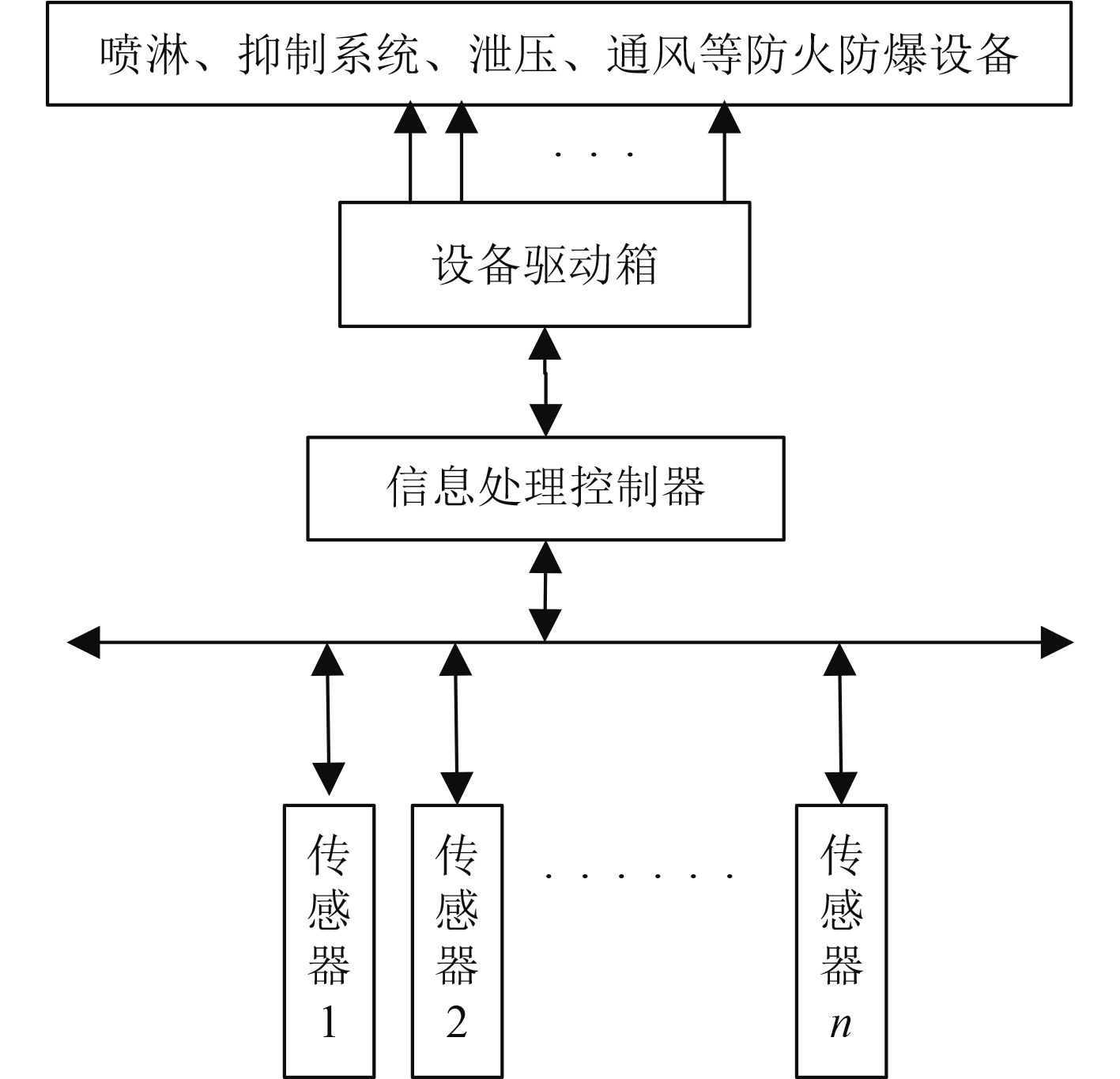图 1 防火防爆安全监控系统结构 Fig. 1 Structure of fire prevention and explosion proof monitoring system

2.2 算法结构模型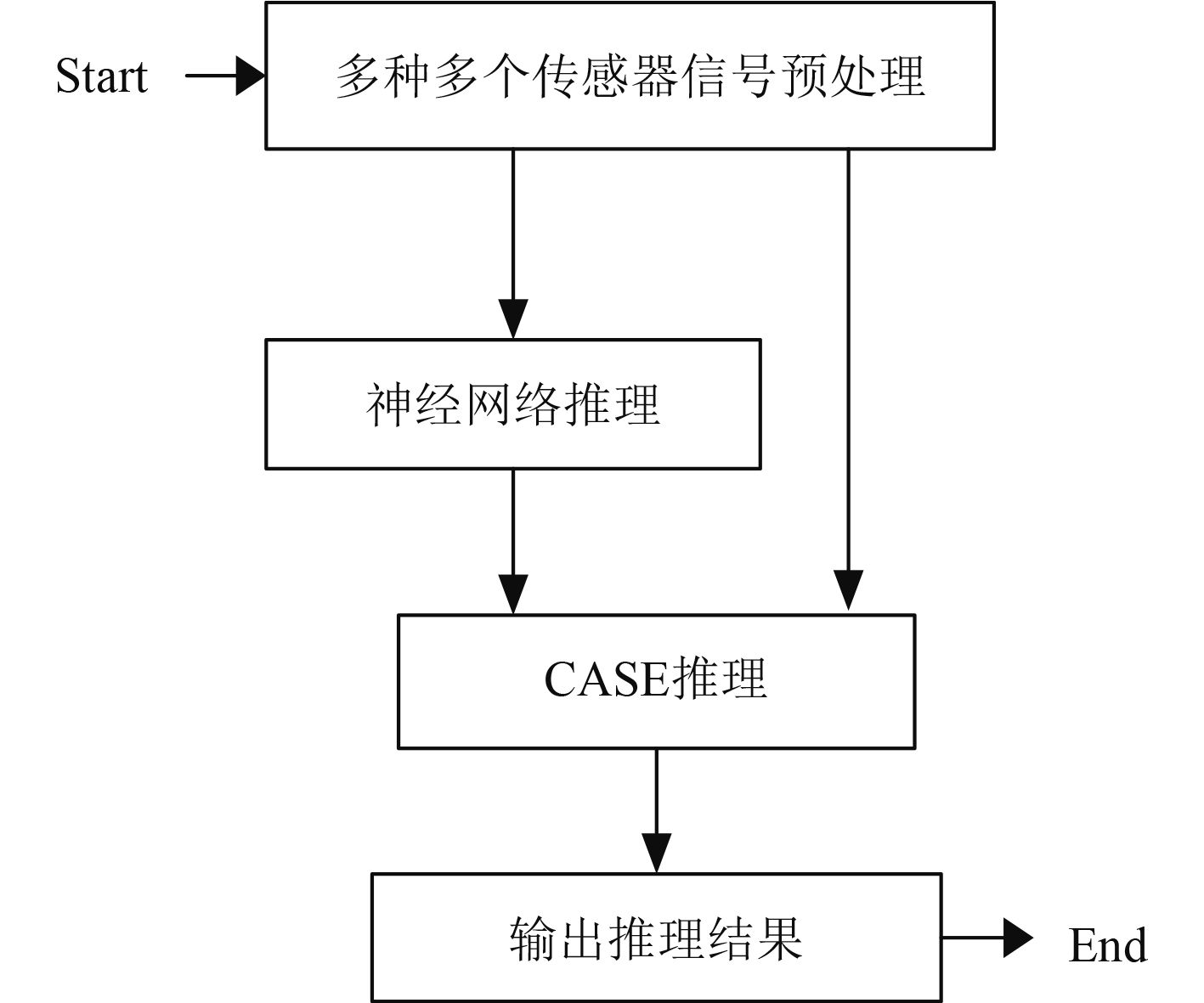图 2 多传感器信息融合算法模型 Fig. 2 Multi-sensor information fusion algorithm model

2.3 传感器信号预处理

 ${a_i}\left( m \right) = \displaystyle\sum\limits_{n = 0}^m {\left( {{x_i}\left( {n + 1} \right) - {x_i}\left( n \right)} \right)} \text{。}$

 ${u_i} = f\left( {{a_i}\left( m \right) - T{h_i}} \right) \text{。}$

2.4 神经网络推理算法

$\displaystyle\frac{{\partial E}}{{\partial Y}} = Y - d$ d为期望输出）

 $\frac{{\partial E}}{{\partial {v_j}}} = \frac{{\partial E}}{{\partial Y}}\frac{{\partial Y}}{{\partial {v_j}}} = \left( {Y - d} \right){O_j} \text{，}$ (1)
 $\frac{{\partial E}}{{\partial {O_j}}} = \frac{{\partial E}}{{\partial Y}}\frac{{\partial Y}}{{\partial {O_j}}} = \left( {Y - d} \right){v_j} \text{，}$ (2)
 ${x_j} = \sum\limits_i {{y_i}{w_{ji}}} \text{，}$ (3)
 ${O_j} = \frac{1}{{1 + {e^{ - x{}_j}}}} \text{，}$ (4)
 $\frac{{\partial E}}{{\partial {w_{ji}}}} = \frac{{\partial E}}{{\partial {O_j}}}\frac{{\partial {O_j}}}{{\partial {w_{ji}}}} \text{，}$ (5)
 $\frac{{\partial {O_j}}}{{\partial {w_{ji}}}} = \frac{{\partial {O_j}}}{{\partial {x_j}}}\frac{{\partial {x_j}}}{{\partial {w_{ji}}}} = {O_j}\left( {1 - {O_j}} \right){y_i} \text{，}$ (6)
 $\frac{{\partial E}}{{\partial {w_{ji}}}} = {O_j}\left( {1 - {O_j}} \right){y_i}\left( {Y - d} \right){v_j} \text{。}$ (7)

2.5 训练数据采集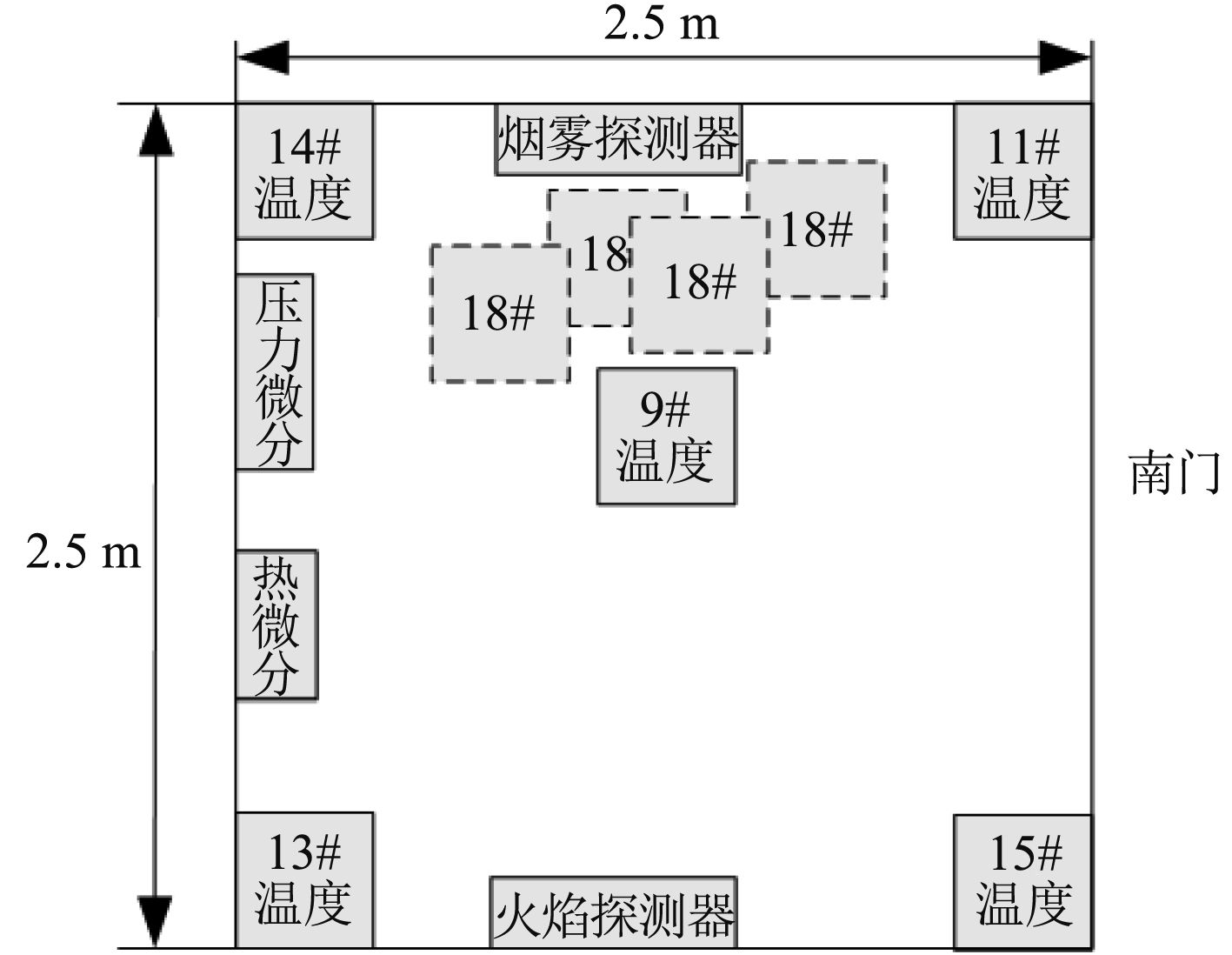图 3 训练数据采集 Fig. 3 Training data acquisition

2.6 数据预处理

DTi（t）=[Ti（t）+Tit-1）+Tit-2）-Tit-3）-Tit-4）-Tit-5）]/3，即求6 s内的平均温度变化率，具有较强的鲁棒性。

F（t）=F0（t）×（F0（t）+F0t-1）×（F0t-1）+F0t-2）×（F0t-2）+F0t-3）×（F0t-3）+F0t-4）×（F0t-4）+F0t-5）））））），F（t）∈[0、6];同理，对烟雾探测器、压力微分探测器、热微分探测器采用相同计算处理，得到：

St）=S0t）×（S0t）+S0t-1）×（S0t-1）+S0t-2）×（S0t-2）+S0t-3）×（S0t-3）+S0t-4）×（S0t-4）+S0t-5））））））；

PDt）=PD0t）×（PD0t）+PD0t-1）×（PD0t-1）+PD0（t-2）×（PD0t-2）+PD0t-3）×（PD0t-3）+PD0t-4）×（PD0t-4）+PD0t-5））））））；

TDt）=TD0t）×（TD0t）+TD0t-1）×（TD0t-1）+TD0t-2）×（TD0t-2）+TD0t-3）×（TD0t-3）+TD0t-4）×（TD0t-4）+TD0t-5））））））；

St），PDt），TDt）∈[0、6]。

T9t），DT9t），T11t），DT11t），T13t），DT13t），T14t），DT14t），T15t），DT15t），Ft），St），PDt），TDt）共14个数据当成一个数据样本发送给监控系统进行处理。

2.7 训练算法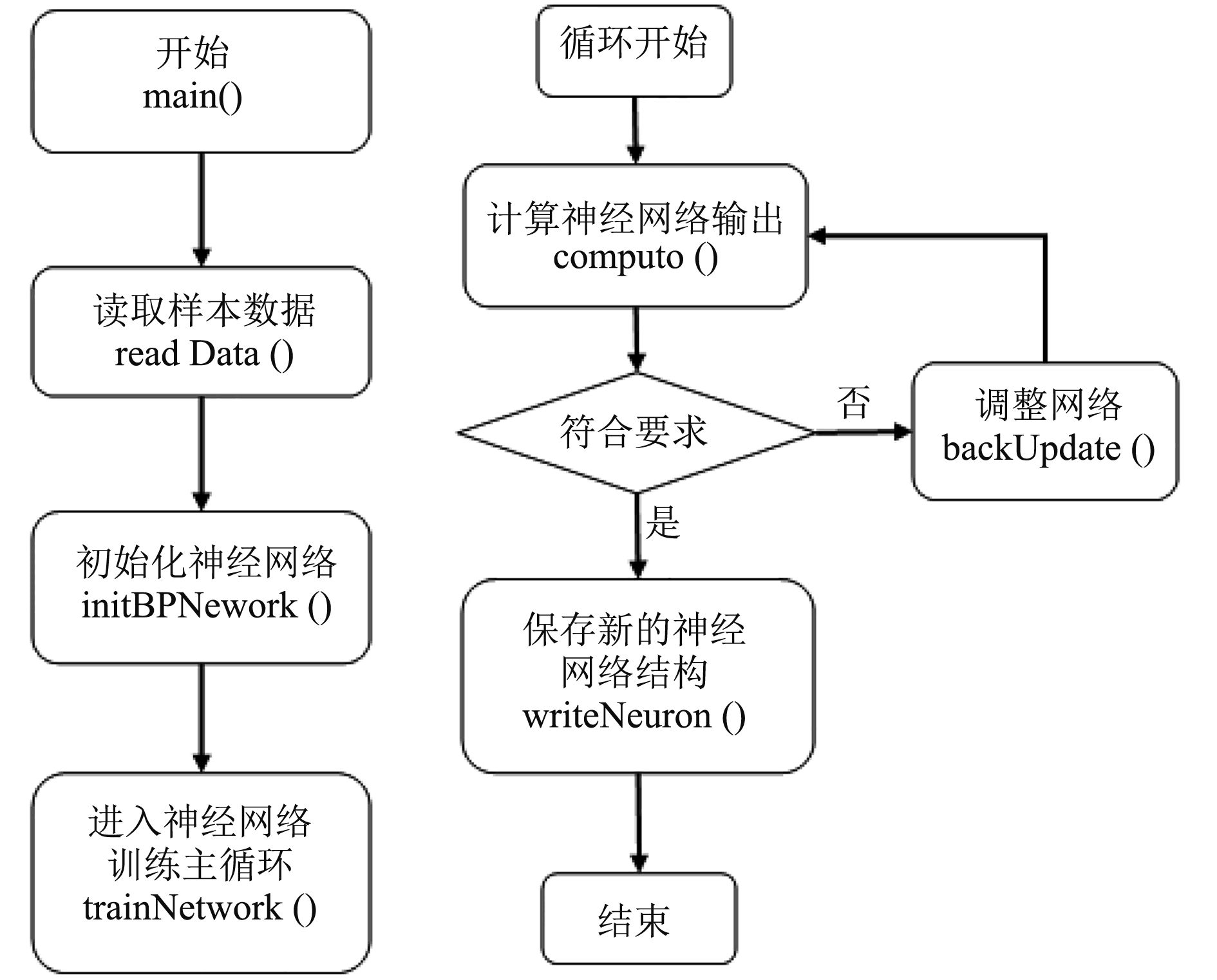图 4 训练系统程序结构图 Fig. 4 Program structure diagram of training system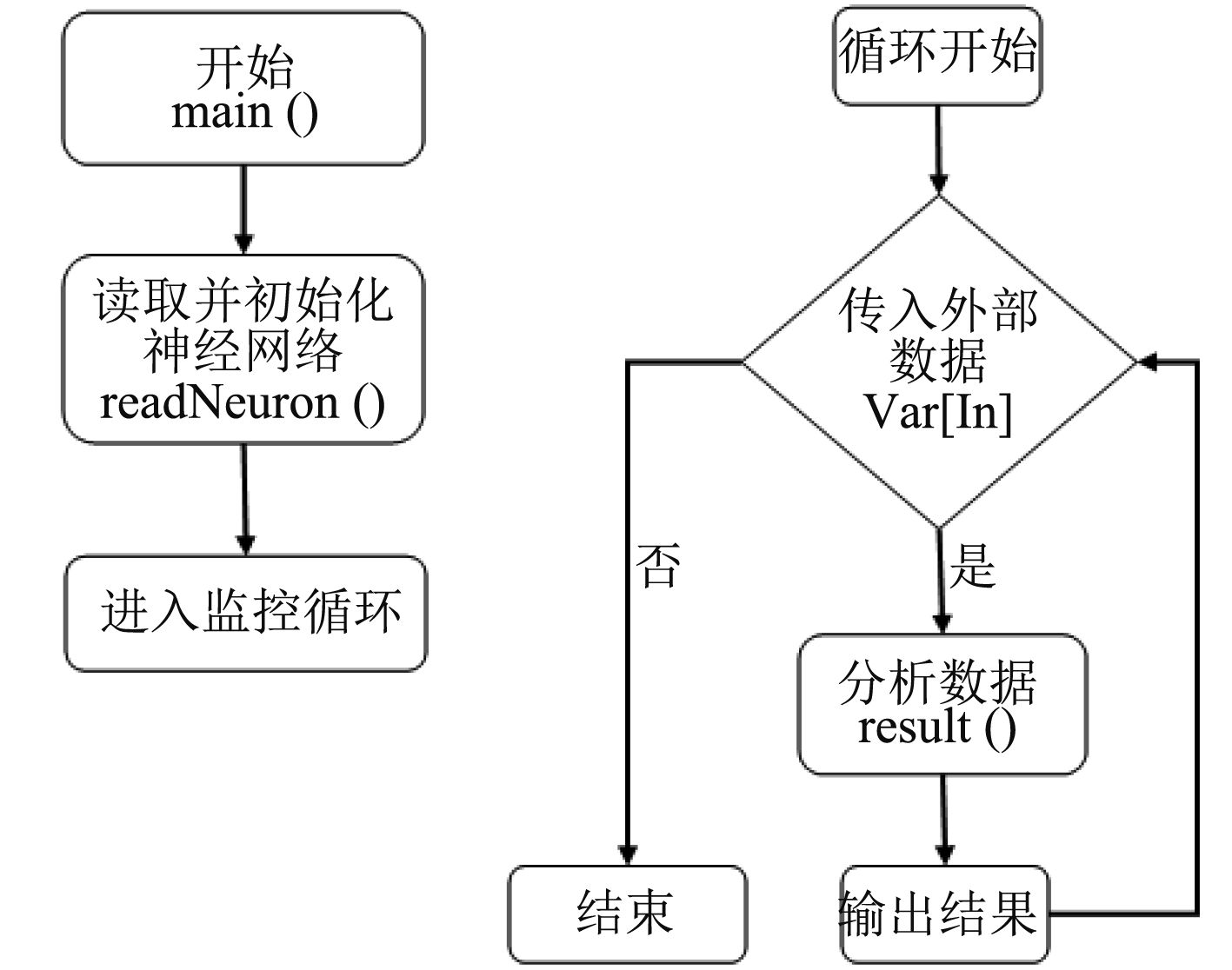图 5 判决系统程序结构图 Fig. 5 Program structure diagram of decision system

3 基于CASE信息库推理算法

3.1 “预警”判决算法

1）温度传感器输出“预警”或“火灾”信号；

2）热微分传感器输出“危险”信号；

3）压力微分传感器输出“危险”信号，或一个压力微分传感器发出“火灾”信号，并且舱室内压力不大于舱外某设定值；

4）火焰传感器输出“危险”信号；

5）烟雾传感器输出“危险”信号；

6）上述状态的组合。

3.2 “火灾”判决算法

1）至少1个温度传感器输出“火灾”信号，且同一弹药舱其他传感器至少输出“预警”；

2）温度微分传感器至少1个发出“火灾”信号；

3）压力微分传感器至少1个发出“火灾”信号，且舱内压力比外部大气压力高过设定值以上；

4）神经网络推理算法输出“火灾”信号，且同一弹药舱内其他传感器输出1个“预警”或“火灾”信号。

4 结　语

  Edward, Multisensor Data Fusion. W, JAMES L. 1990.  THOMAS J M, JAMES M, TEKIN A K. Using multivariate statistical methods to detect fire[J]. Fire Technology. 1996. 32(1): 23–29.  JAMES A M. Using multiple sensors for discriminating fire detection[J]. Fire Suppression and Research Application Symposium. Orlando FL. USA.l999: pp 150–164.  康耀红. 数据融合理论与应用 [M]. 西安: 西安电子科技大学出版社 1997.  何友. 多传感器信息融合及应用[M]. 北京: 电子工业出版社, 2000. 11.  藤召胜, 罗隆福, 童调生. 智能检测系统与数据融合[M]. 北京: 机械工业出版社, 1999. 12.  王丽萍, 基于多传感器信息融合技术的火灾探测系统研究[D]. 湖南: 湖南大学, 2006. WANG Li-ping, Research on fire detection system based on multisensor information fusion technology[D]. Hunan: Hunan University, 2006.